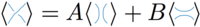# 06-1350/Class Notes for Thursday September 14

The Mathematica notebook we wrote is here.

### Jones Polynomial

The simplest way to define the Jones polynomial is via the Kauffman bracket. The idea is to eliminate all crossings using the rule:In the right hand side, the first bracket is called the 0-smoothing and the second is called the 1-smoothing. To calculate the Kauffman bracket we must sum over all possible smoothings. For instance, for the trefoil, we 23 = 8 summands, one of which will be:

Each summand will have no crossing and thus will be a union of (possibly nested) unknots. We define the bracket polynomial of k unknots to be dk-1 for some indeterminate d. Our hopes that our polynomial in ℤ[d,A,B] will be an invariant under the Reidmeister moves. We first verify R2.

Collecting like terms and comparing we find AB = 1 and A2 + B2 + dAB = 0. Thus, we must have B = A-1 and d = -(A2 + A2). Things are looking bad, we still have two moves to verify and we already lost two of our variables.

We now verify R3. For this we remark that

The two diagrams with coefficients B coincide; and the diagrams with coefficients A differ by two R2 moves. Now we verify R1:

The right hand side evaluates to A + A-1(-A2 -A-2) = -A3 of the desired. This is unfortunate. One could salvage something by taking A to be one of the cube roots of -1; however, the right way out of this is to define another invariant which fails in the exactly the same way, and multiply it with the bracket polynomial.

### Writhe

The invariant we are looking for is called the writhe. If D is a diagram of an oriented knot, we define

We choose +1 if the crossing is positive (the overpass goes over the underpass from left to right) and -1 if the crossing is negative (otherwise):

Lets have an example. Notice that the orientation of the knot is actually irrelevant.

We now show that the writhe is also invariant under R2 and R3, under R1 we gain ±1 depending which way we apply it. In R2, the two crossings have opposite signs; and in R3, the diagonal crossing doesn’t change sign and the other two are reversed.

It follows that 〈D〉⋅(-A-3)w(d) is a knot invariant. This is a polynomial in A, we now substitute q http://katlas.math.toronto.edu/drorbn/images/2/23/Mathtext2.png for A and call this the Jones polynomial (which strictly speaking, is not really a polynomial).